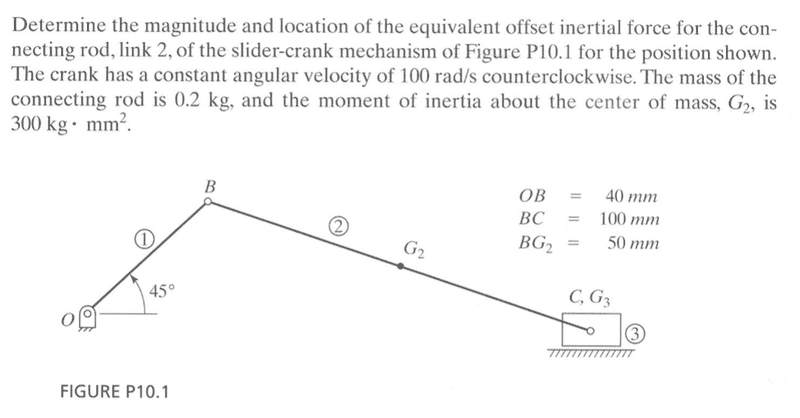# Inertial forces of a slider crank

Jstuff

## Homework StatementHello, I have an exam later in the week and I'm trying to understand how to this problem.

Sum of forces.
Sum of moments.

## The Attempt at a Solution

I broke the mechanism into 3 pieces, the crank, connecting rod, and slider and then began to analyze each piece with the sum of moments and sum of forces on each. It seems that I am missing too many unknowns. For example on this sum of forces on crank what is the mass and acceleration of the crank.

Thanks in advance for the help.[/B]